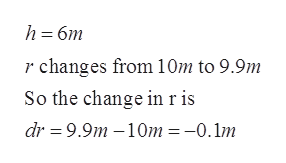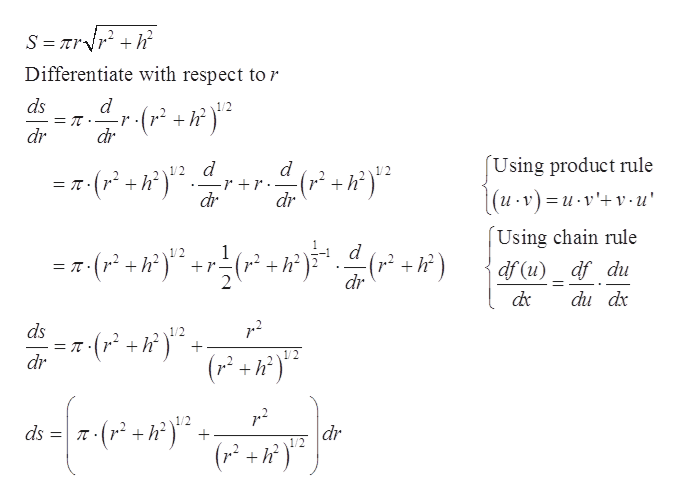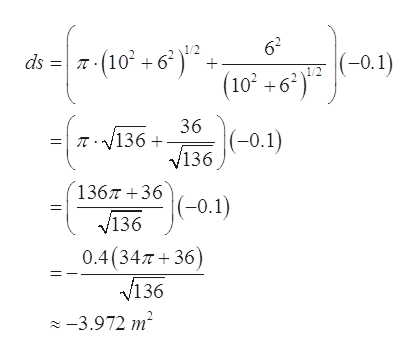# Linear Approximate the change in the lateral surface area (excluding the area of the base) of a right circular cone with a fixed height h=6m when its radius decreases from r=10m to r=9.9m (S=pi x r (square root of r2 +h2)

Question
12 views

Linear Approximate the change in the lateral surface area (excluding the area of the base) of a right circular cone with a fixed height h=6m when its radius decreases from r=10m to r=9.9m (S=pi x r (square root of r+h2)

check_circle

Step 1

We havehelp_outlineImage Transcriptioncloseh 6m r changes from 10m to 9.9m So the change in ris dr 9.9m-10m =-0.1m fullscreen
Step 2

First find the differentiation of lateral surface area with respect to r.help_outlineImage TranscriptioncloseDifferentiate with respect tor ds -r (r2 +h) dr dr (Using product rule d d 2 h2 : (r* +h (и -т) —и-v'+v-u" dr dr Using chain rule d 1 1/2 df (u)_ df du = T-r h* r dr du dx ds dr (r+h dr h fullscreen
Step 3

Now substitute dr = – 0.1...help_outlineImage Transcriptionclose62 ds (10+6 1/2 (-0.1) (10 +6 36 (-0.1) v136 V136 136736 (-0.1) 0.4(347 36) v136 2 -3.972 m2 fullscreen

### Want to see the full answer?

See Solution

#### Want to see this answer and more?

Solutions are written by subject experts who are available 24/7. Questions are typically answered within 1 hour.*

See Solution
*Response times may vary by subject and question.
Tagged in
MathCalculus

### Derivative